﻿ SQUARES IN VEDIC MATHEMATICS | SHORTCUT METHOD FOR SQUARE ANY NUMBER #EDUCRATSWEB
Guest Post | Submit   Job information   Contents   Link   Youtube Video   Photo   Practice Set   Affiliated LinkRegisterLoginJoin Our Telegram Group https://t.me/educratsweb

### Squares in Vedic Mathematics | Shortcut method for Square any number

Posted By educratsweb.comStudy Material 🗓 Sunday November 17 2019 👁 318

Tirthaji Maharaj has mentioned tricks to Square Numbers in Vedic Mathematics in Specific and General Methods. Specific Multiplication Methods can be applied when numbers satisfy certain conditions like number ending with 5 or number closer to power of 10, etc. While General Multiplication Methods can be applied to any type of number.

Depending on Specific and General Techniques, Squares in Vedic Mathematics are classified in the form of Sutras as below. Lets see the  Vedic Mathematics Squares shortcut techniques.

Calculating Vedic Math Square Tricks can be classified in following types:

3. DvandaYoga (General Method)

It is a specific and shorcut to square numbers using Vedic Mathematics whenever number is closer to power of 10. (10, 100, 1000, ….)

Lets see examples for  vedic maths square method of Yavadunam:

Square of 14:

142 = (14+4)/42 = 18/16  = 196

Here 14 is 4 more than 10(Base 10), So Excess = 4
Increase it still further to that extent, So (14+4) = 18
Square its excessive, So 42= 16

Square of 97:

972 = (97-3)/32 = 94/09  = 9409

Here 97 is 3 less than 100(Base 100), So deficiency =3
Reduce it still further to that extent, So (97-3) = 94.
Square its deficiency, So 32 = 09. (As base is 100, we need exactly 2 digits. Hence 09).

This is another specific� vedic maths tricks for square of a number ending with 5.

Lets see examples for vedic squares tricks using Ekadhikena Purvena.

1. Check if last digit is 5, if yes – square of 5 is 25
2. Apply Ekadhikena Purvena for rest of the number i.e. Add 1 to the previous number and multiply each other. Example in case of square of 85, Add 1 to 8 to get 9 and multiply this with 8.
3. Steps 1 and 2 together gives final answer.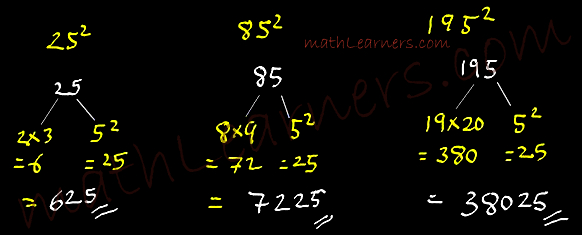## DvandaYoga (Duplex Method):

Dvanda Yoga is general method to square any number in vedic maths. Dvanda Yoga or Duplex Method is shortcut method for squares of large numbers.

How to calculate Dvanda

Concept:

D(3) = 32 = 9
D(43) = 2x4x3 = 24
D(567) = 2x5x7 + 62 = 70 + 36 = 106
D(3456) = 2x3x6 + 2x4x5 = 36 + 40 = 76
D(34567) = 2x3x7 + 2x4x6 + 52 = 42 + 48 + 25 = 115

Example:वृश्चिक राशी के गुण और अवगुण /वृश्चिक राशी /vrischik rashi /scorpius character......by Vedic AstroLearn Vedic Astrology Step by Step Lesson 1 by Sundeep Kataria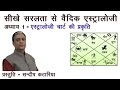Hindi Learn Vedic Astrology Lesson 1 by Sundeep Kataria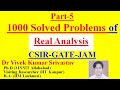Part-5:Real Analysis: (41-50) Ques/Study Material for CSIR/NET/GATE/JAM/B.Sc./M.Sc./Mathematics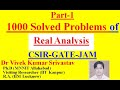Part-1-Real Analysis: (1-10) Ques/Study Material for CSIR/NET/GATE/JAM/B.Sc./M.Sc./MathematicsDurga Puja 2019 Kolkata | 20 Crore Gold Durga | Santosh Mitra Square | Durga Pujo 2019 Pandal Making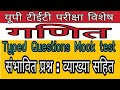UP TET EXAM SPECIAL: MATHEMATICS MOCK TEST WITH EXPLANATION BY GYANबिना पेन उठाये Calculation कैसे करे (Vedic Maths, Digit Sum) for SSC, BANK, CATAptitude Made Easy - Problems on Alligation and Mixture Part-1 – Basics and Methods,Aptitude tricks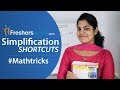Aptitude made easy- Simplification Shortcuts 1 - SSC,Railway,IBPS #Mathtricks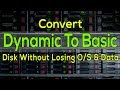[100% Solved Without Losing Data] Easily Convert Dynamic Disk To Basic Disk | Best Methodकार्यपालक सहायक परीक्षा (Executive Assistant Exam) सफलता के टिप्स नंबर 1 | Shortcut KeyAptitude Made Easy - Compound Interest 2 – Basics and Methods, Exam Questions, Math tricks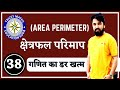Mesuration square part 1|| TRICKY Maths Ak choudhary ||ssc RAILWAY bank IBPS Rajasthan Police